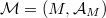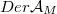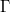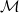﻿

#### Abstract

Suppose that${\mathcal{M}}=(M,{\mathcal A}_M)$ is a graded manifold and consider a direct subsheaf$\cd$ of${ Der {\mathcal A}_M}$ and a graded vector field$\Gamma$ on${\mathcal{M}}$, both satisfying certain conditions.$\cd$ is used to characterize the local expression of$\Gamma$. Thus we review some of the basic definitions, properties, and geometric structures related to the theory of adjoint symmetries on a graded manifold and discuss some ideas from Lagrangian supermechanics in an informal fashion. In the special case where${\mathcal{M}}$ is the tangent supermanifold, we are able to find a generalization of the adjoint symmetry method for time-dependent second-order equations to the graded case. Finally, the relationship between adjoint symmetries of$\Gamma$ and Lagrangians is studied.

DOI Code: 10.1285/i15900932v39n1p33

Keywords: supermanifold; involutive distribution; second-order differential equation field; Lagrangian systems; adjoint symmetry

Full Text: PDF